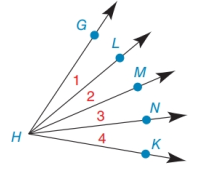Chapter 1.6, Problem 26E### Elementary Geometry for College St...

6th Edition
Daniel C. Alexander + 1 other
ISBN: 9781285195698

#### Solutions

Chapter
Section### Elementary Geometry for College St...

6th Edition
Daniel C. Alexander + 1 other
ISBN: 9781285195698
Textbook Problem
1 views

#The Angle-Addition Postulate can be generalized as follows: “The measure of an angle equals the sum of measures of its parts.” State a general conclusion regarding m ∠ G H K based on the figure shown.

To determine

To state:

The conclusion for mGHK according to the given figure.

Explanation

Given:

The given figure is,

Approach:

The Angle-Addition Postulate is defined as,

“The measure of an angle equals the sum of measures of its parts.”

Calculation:

From the given figure, it can be noticed that point L, M and N divides angle mGHK in four equal parts.

According to the Angle-Addition Postulate the measure of GHK is,

mGHK=mGHL+mLHM+mMHN+mNHK

Substitute 1 for

### Still sussing out bartleby?

Check out a sample textbook solution.

See a sample solution

#### The Solution to Your Study Problems

Bartleby provides explanations to thousands of textbook problems written by our experts, many with advanced degrees!

Get Started